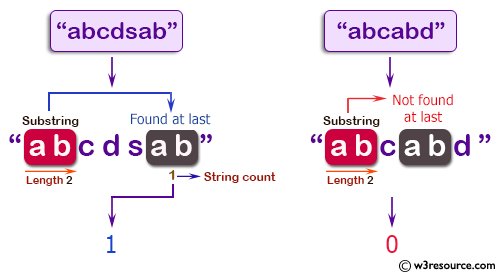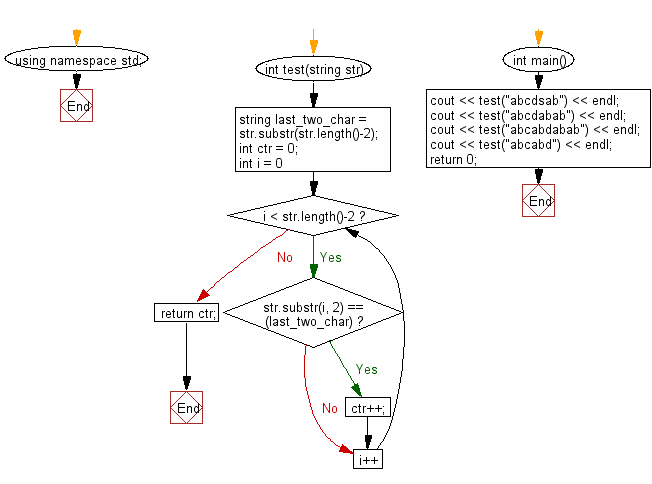﻿ C++ : Count a substring of length 2 appears in a string# C++ Exercises: Count a substring of length 2 appears in a given string and also as the last 2 characters of the string

## C++ Basic Algorithm: Exercise-30 with Solution

Write a C++ program to count a substring of length 2 appears in a given string and also as the last 2 characters of the string. Do not count the end substring.

Sample Solution:

C++ Code :

``````#include <iostream>
using namespace std;

int test(string str)
{
string last_two_char = str.substr(str.length()-2);
int ctr = 0;

for (int i = 0; i < str.length()-2; i++)
{
if (str.substr(i, 2) == (last_two_char)) ctr++;
}
return ctr;
}

int main()
{
cout << test("abcdsab") << endl;
cout << test("abcdabab") << endl;
cout << test("abcabdabab") << endl;
cout << test("abcabd") << endl;
return 0;
}
``````

Sample Output:

```1
2
3
0
```

Pictorial Presentation:Flowchart:C++ Code Editor:

Contribute your code and comments through Disqus.

What is the difficulty level of this exercise?

﻿